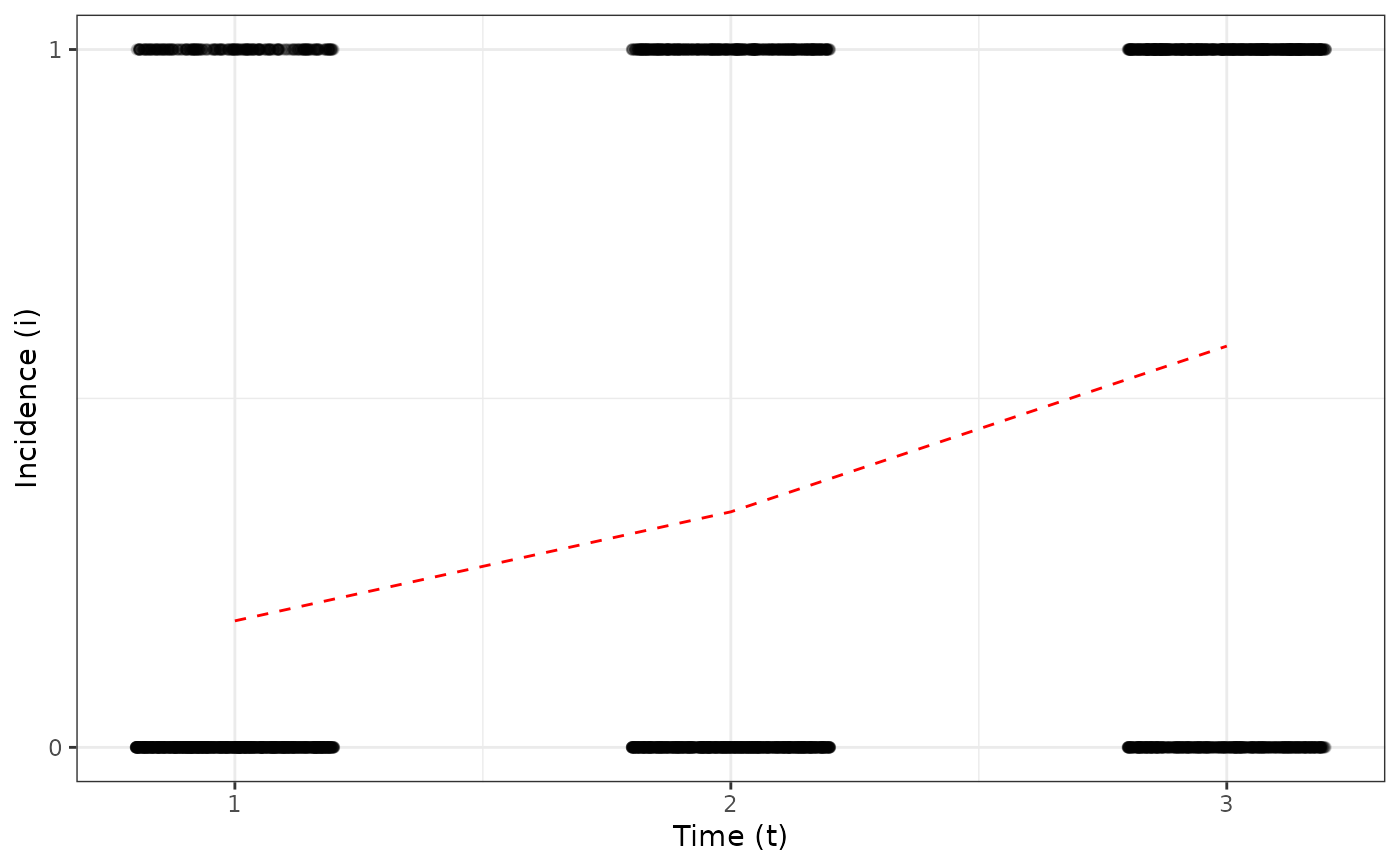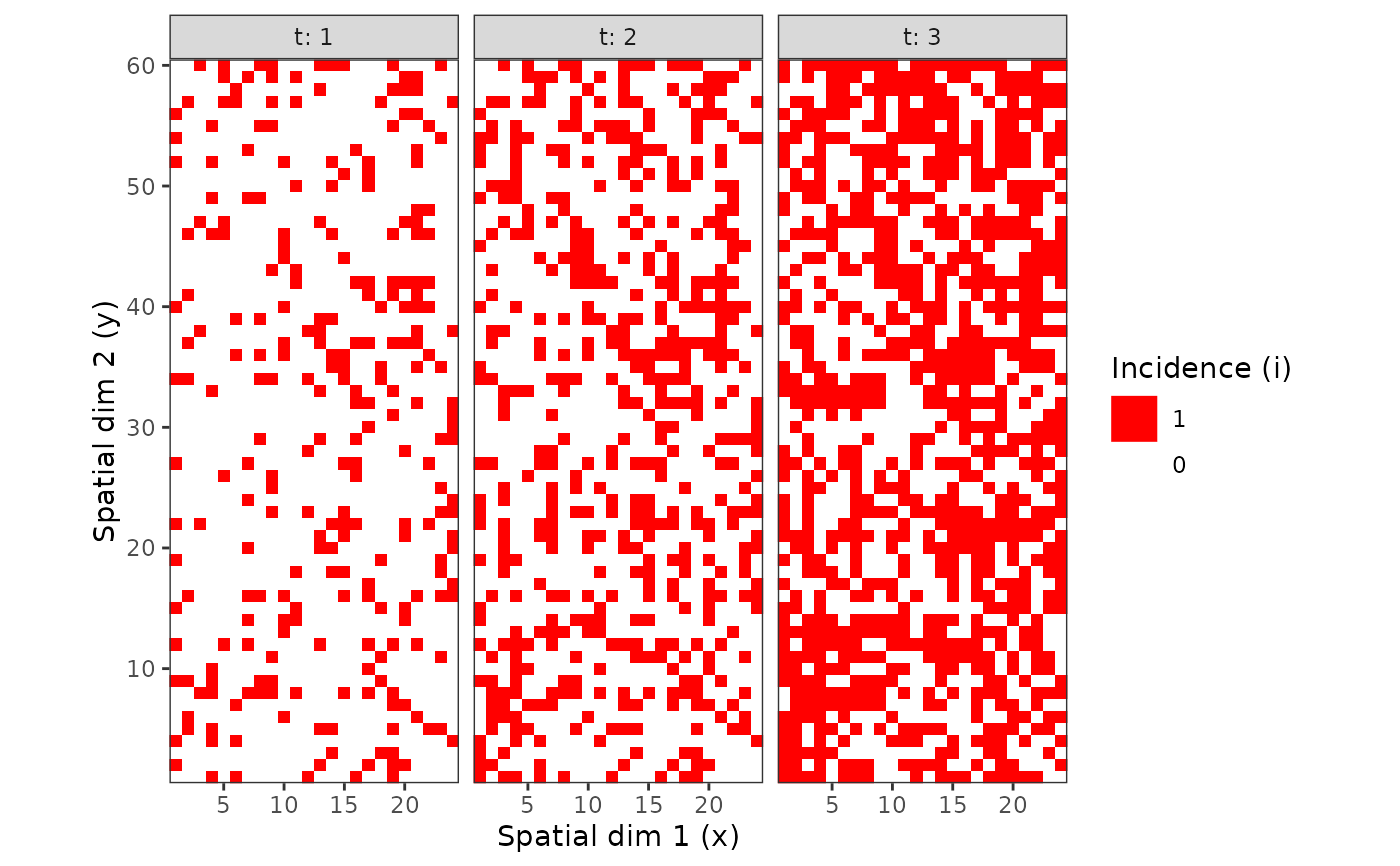Divide into groups and reassemble.

## Usage

# S3 method for intensity
split(x, f, drop = FALSE, ..., by, unit_size)

## Arguments

x

vector or data frame containing values to be divided into groups.

f

a ‘factor’ in the sense that as.factor(f) defines the grouping, or a list of such factors in which case their interaction is used for the grouping. If x is a data frame, f can also be a formula of the form  ~ g to split by the variable g, or more generally of the form  ~ g1 + ... + gk to split by the interaction of the variables g1, ..., gk, where these variables are evaluated in the data frame x using the usual non-standard evaluation rules.

drop

logical indicating if levels that do not occur should be dropped (if f is a factor or a list).

...

further potential arguments passed to methods.

by

The name(s) of the variable(s) which define(s) the grouping.

unit_size

Size of a group unit. It must be a named vector, with names corresponding to non-observational variables (i.e. space and time variables). If the size of a variable in the data set is not a multiple of the provided value in unit_size, some sampling units (the last ones) will be dropped so that clumps of individuals remain even throughout the data set.

## Value

A list of intensity objects.

## Examples

my_incidence <- incidence(tomato_tswv\$field_1929)
plot(my_incidence, type = "all")
#> Warning: Computation failed in stat_summary()
#> Caused by error in get():